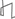Research Abstracts Home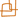CSAIL Digital Archive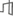Research Activities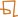CSAIL HomeResearch Abstracts - 2007IntroductionPerception & LearningPhysical, Biological & Social SystemsSystemsTheory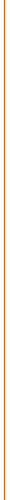### Computational Geometry through the Information Lens

#### Erik D. Demaine & Mihai Pătraşcu

##### Overview

We are revisiting classic problems in computational geometry from the modern algorithmic perspective of exploiting the bounded precision of the input. In one dimension, this viewpoint has taken over as the standard model of computation, and has led to a powerful suite of techniques for both upper and lower bounds that constitute a mature field of research. But the same tools simply do not apply to two and higher dimensions, aside from orthogonal problems. For example, it is tempting to wonder whether the simple idea of radix sort can be extended to improve the running time of a simple two-dimensional problem like constructing a Voronoi diagram, but this problem has remained uncracked for many years despite extensive effort.

We aim to fill this gap between computational geometry, which generally assumes infinite precision inputs and computation, and most of the rest of theoretical computer science. This gap has become increasingly apparent as techniques for finite-precision one-dimensional problems have been improved and developed. For this reason, several researchers have exploited finite precision in orthogonal problems in higher dimensions, where essentially the same one-dimensional ideas apply, but have so far been unsuccessful in extending these results to nonorthogonal problems. This shortcoming for nonorthogonal problems was highlighted 15 years ago by Willard  and repeatedly since.

##### Our Results

We have jump-started this direction of research with several recent papers:

• In FOCS 2006, we  develop the first sublogarithmic bound for planar point location queries. Specifically, our linear-space static data structure supports queries in O(min{lg n / lg lg n, √w / lg w}) time, where w is the number of bits of precision (word size). This result also constitutes the first sublogarithmic bound for exact nearest neighbors in the plane. Similar results were independently discovered by Chan , which has led to subsequent collaboration.
• In SoCG 2007, we  developed the first dynamic data structure for a nonorthogonal geometric problem with sublogarithmic query time. Specifically, we show how to maintain the convex hull of a set of n points in polylogarithmic update time and O(lg n / lg lg n) query time. We also prove a matching lower bound.
• In STOC 2007, we  develop an even faster algorithm for offline planar point location, running in time n ⋅ 2O(√lg lg n), which is o(n lgε n) for all ε > 0. This basic result enables many fundamental algorithmic problems to be solved in the same time bound: Voronoi diagram construction, Delaunay triangulation, 3D convex hull, line-segment intersection reporting, triangulating a polygon with holes, largest empty circle, Euclidean minimum spanning tree, etc.
• In Algorithmica , we develop the first subquadratic algorithms for 3SUM, a problem which plays an important role in understanding geometric problems that seem to require superlinear polynomial time.
##### Discussion

There is a close relation between this direction of research and engineering practice. Geometric computer programs already exploit the fixed precision of the input, and our work can be seen as a justification for some of these “heuristics”. Our goal is to understand the extent to which fixed precision can be exploited and to develop new, more practical techniques for solving classic geometric problems.

Our work so far has forced us to re-analyze many “standard” ideas and techniques in geometry through a new, information-theoretic lens. This view of geometry seems interesting in its own right, and the algorithmic puzzles it raises are very appealing.

##### References:

 Dan E. Willard. Applications of the fusion tree method to computational geometry and searching. In Proceedings of the 3rd Annual ACM-SIAM symposium on Discrete Algorithms, pp. 286–295, Orlando, Florida, United States, 1992.

 Mihai Pătraşcu. Planar point location in sublogarithmic time. In Proceedings of the 47th Annual IEEE Symposium on Foundations of Computer Science, pp. 325–332, Berkeley, California, October 2006.

 Timothy M. Chan. Point location in o(log n) time, Voronoi diagrams in o(n log n) time, and other transdichotomous results in computational geometry. In Proceedings of the 47th Annual IEEE Symposium on Foundations of Computer Science, pp. 333–342, Berkeley, California, October 2006.

 Erik D. Demaine and Mihai Pătraşcu. Tight bounds for dynamic convex hull queries (again). In Proceedings of the 23rd Annual ACM Symposium on Computational Geometry, Gyeongju, South Korea, June 2007. To appear.

 Timothy M. Chan and Mihai Pătraşcu. Voronoi diagrams in n ⋅ 2O(√lg lg n) time. In Proceedings of the 39th ACM Symposium on Theory of Computing, San Diego, California, June 2007. To appear.

 Ilya Baran, Erik D. Demaine, and Mihai Pătraşcu. Subquadratic algorithms for 3SUM. Algorithmica. To appear.Computer Science and Artificial Intelligence Laboratory (CSAIL) The Stata Center, Building 32 - 32 Vassar Street - Cambridge, MA 02139 - USA tel:+1-617-253-0073 - publications@csail.mit.edu